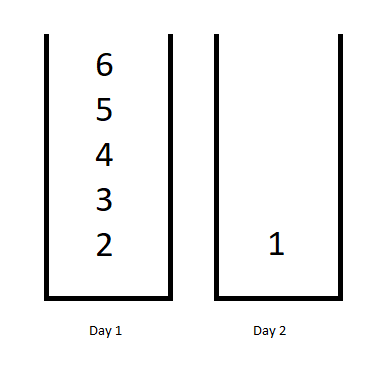# 1335. Minimum Difficulty of a Job Schedule

You want to schedule a list of jobs in `d` days. Jobs are dependent (i.e To work on the `i-th` job, you have to finish all the jobs `j` where `0 <= j < i`).

You have to finish at least one task every day. The difficulty of a job schedule is the sum of difficulties of each day of the `d` days. The difficulty of a day is the maximum difficulty of a job done in that day.

Given an array of integers `jobDifficulty` and an integer `d`. The difficulty of the `i-th` job is `jobDifficulty[i]`.

Return the minimum difficulty of a job schedule. If you cannot find a schedule for the jobs return -1.

Example 1:```Input: jobDifficulty = [6,5,4,3,2,1], d = 2
Output: 7
Explanation: First day you can finish the first 5 jobs, total difficulty = 6.
Second day you can finish the last job, total difficulty = 1.
The difficulty of the schedule = 6 + 1 = 7
```

Example 2:

```Input: jobDifficulty = [9,9,9], d = 4
Output: -1
Explanation: If you finish a job per day you will still have a free day. you cannot find a schedule for the given jobs.
```

Example 3:

```Input: jobDifficulty = [1,1,1], d = 3
Output: 3
Explanation: The schedule is one job per day. total difficulty will be 3.
```

Example 4:

```Input: jobDifficulty = [7,1,7,1,7,1], d = 3
Output: 15
```

Example 5:

```Input: jobDifficulty = [11,111,22,222,33,333,44,444], d = 6
Output: 843
```

Constraints:

• `1 <= jobDifficulty.length <= 300`
• `0 <= jobDifficulty[i] <= 1000`
• `1 <= d <= 10`

Hard

Normal

Turvo

### Solution(Chinese):

LEETCODE 1335. Minimum Difficulty of a Job Schedule 解题思路分析